### IMO Shortlist 2015 problem N4

Kvaliteta:
Avg: 0,0
Težina:
Avg: 7,0

Suppose that$a_0, a_1, \cdots$ and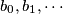$b_0, b_1, \cdots$ are two sequences of positive integers such that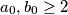$a_0, b_0 \ge 2$ and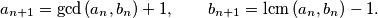Show that the sequence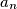$a_n$ is eventually periodic; in other words, there exist integers$N \ge 0$ and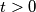$t > 0$ such that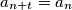$a_{n+t} = a_n$ for all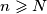$n \geqslant N$.

(France)

Izvor: https://www.imo-official.org/problems/IMO2015SL.pdf